• 需要自行下载matrix.h文件，可以直接运行，得出插值估计值与实际值比较的结果。rbf
• 只不过在显示二维的数据插值的过程中，需要meshgrid函数产生数据，进行显示插入数据的输出值。 产生二维数据： clear all; figure; %**************************************************************************...
二维数据的插值过程跟一维数据的过程一样，
只不过在显示二维的数据插值的过程中，需要meshgrid函数产生数据，进行显示插入数据的输出值。
产生二维数据：
clear all; figure; %************************************************************************** % 2D Interpolation %************************************************************************** %Matlab standard interpolation using griddata  rand('seed',0) x = rand(50,1)*4-2; y = rand(50,1)*4-2; z = x.*exp(-x.^2-y.^2);
用matlab自带的插值函数进行插值 ti = -2:.05:2;  [XI,YI] = meshgrid(ti,ti); ZI = griddata(x,y,z,XI,YI,'cubic');   subplot(2,2,1); mesh(XI,YI,ZI), hold, axis([-2 2 -2 2 -0.5 0.5]);  plot3(x,y,z,'.r'), hold off; title('Interpolation using Matlab function griddata(method=cubic)');   subplot(2,2,3); pcolor(abs(ZI - XI.*exp(-XI.^2-YI.^2))); colorbar; title('griddata(method=cubic) interpolation error');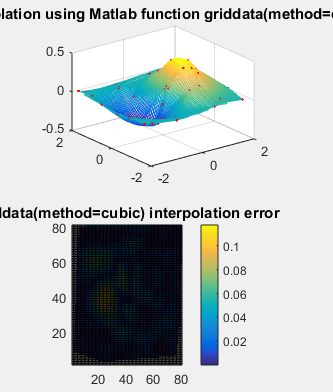现在使用径向基函数的插值方法： 过程跟一维的数据一样
% 2D Interpolation %************************************************************************** %Matlab standard interpolation using griddata  rand('seed',0) x = rand(50,1)*4-2; y = rand(50,1)*4-2; z = x.*exp(-x.^2-y.^2);   ti = -2:.05:2;  [XI,YI] = meshgrid(ti,ti); ZI = griddata(x,y,z,XI,YI,'cubic');   subplot(2,2,1); mesh(XI,YI,ZI), hold, axis([-2 2 -2 2 -0.5 0.5]);  plot3(x,y,z,'.r'), hold off; title('Interpolation using Matlab function griddata(method=cubic)');   subplot(2,2,3); pcolor(abs(ZI - XI.*exp(-XI.^2-YI.^2))); colorbar; title('griddata(method=cubic) interpolation error'); %% %RBF interpolation %op=rbfcreate([X(:)'; Y(:)'], Z(:)','RBFFunction', 'thinplate'); rbfcheck(op); %op=rbfcreate([X(:)'; Y(:)'], Z(:)','RBFFunction', 'linear'); rbfcheck(op); %op=rbfcreate([X(:)'; Y(:)'], Z(:)','RBFFunction', 'cubic'); rbfcheck(op); %op=rbfcreate([X(:)'; Y(:)'], Z(:)','RBFFunction', 'gaussian'); rbfcheck(op); op=rbfcreate([x'; y'], z','RBFFunction', 'multiquadric', 'RBFConstant', 2); rbfcheck(op); %op=rbfcreate(x, y,'RBFFunction', 'gaussian'); %op=rbfcreate(x, y); ZI = rbfinterp([XI(:)'; YI(:)'], op);   ZI = reshape(ZI, size(XI)); subplot(2,2,2); mesh(XI,YI,ZI), hold plot3(x,y,z,'.r'), hold off; title('RBF interpolation'); axis([-2 2 -2 2 -0.5 0.5]); subplot(2,2,4); pcolor(abs(ZI - XI.*exp(-XI.^2-YI.^2))); colorbar; title('RBF interpolation error');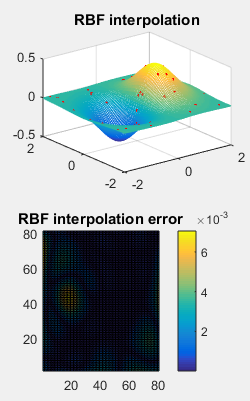展开全文•RBF
• 该方法通过建立图像的灰度一梯度直方图获取聚类数目，作为差分演化算法(DE)问题的维数，结合改进的FCM自适应提取图像破损区域，在此基础上，利用径向基函数插值方法fRBF)对图像进行修复．经实验验证，该方法能解决...
• 将ENO格式和径向基函数插值相结合，提出了求解双曲型偏微分方程的径向基函数插值的ENO方法。该方法依据ENO思想建立自适应模板，在选定的模板上利用径向基函数进行逼近，能够很好地处理具有间断解的问题，消除间断点...
• 曲面插值我们一般使用 径向基插值的方法： RBF (Radial Basis Function)可以看作是一个高维空间中的曲面拟合(逼近)问题，学习是为了在多维空间中寻找一个能够最佳匹配训练数据的曲面，然后来一批新的数据，用刚才...

当我们遇到高维的数据，二维或者三维的数据时，由于数据的不完整，需要预测一些数值，一般我们的方法是用曲面重构，
曲面重构可分为：1、插值2、逼近
曲面插值我们一般使用 径向基插值的方法：

RBF 方是一系列精确插值方法的组合；即表面必须通过每一个测得的采样值。有以下五种基函数：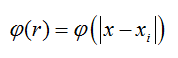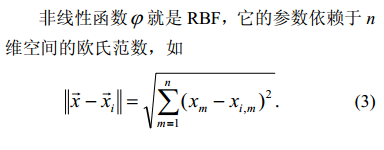1、Gaussian  高斯曲面函数3、linear:线性函数4、cubic:立方体曲面函数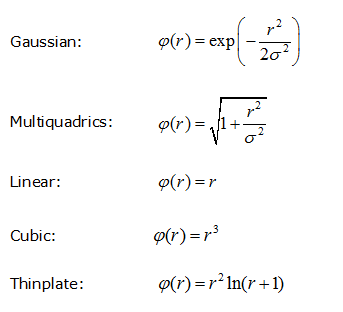径向基函数的插值函数表达式：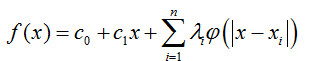现在我们的目的是根据已知的N个数据，求出函数f(x)的系数  。。。。。。。。C0, C1 and λi。。。。。。。。。。
其中xi是已知的数据点集，

而我们这里得到这些未知参数的方法主要是：最小二乘法。。。。或者SVD
假设我们有N组数据集，以及对应的函数f(xi)的值，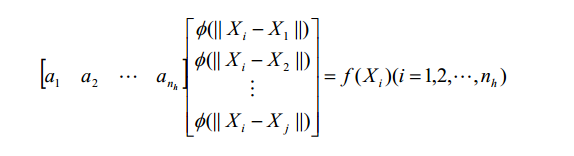这样由N组数据我们可以一个矩阵：A *B=Y
其中A是未知参数矩阵，B是数据集得到的值，Y是数据集对应的输出值，
我们可以用最小二乘法得到参数A的值。。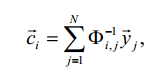代码下载地址：
点击打开链接

展开全文• 根据界面能量守恒原理，将径向基函数（RBF）引入流固耦合分析领域，提出了基于RBF插值的流固耦合界面数据传递方法（RBF/FSI）。推导了界面位移传递矩阵，并根据RBF/FSI算法编制了相应的界面信息传递的计算程序。以三...
• 基于径向基函数局部插值的加权最小二乘法,给出了方法的基本原理及求解的一般过程。通过对 Poisson方程和悬臂梁弯曲的计算验证了该方法的有效性,并讨论了几个关键因素对收敛性的影响,得出了一 些有益的结论。
• 只不过在显示二维的数据插值的过程中，需要meshgrid函数产生数据，进行显示插入数据的输出值。 产生二维数据： clear all; figure; %************************************************************************** %...
二维数据的插值过程跟一维数据的过程一样，
只不过在显示二维的数据插值的过程中，需要meshgrid函数产生数据，进行显示插入数据的输出值。
产生二维数据：

clear all; figure;
%**************************************************************************
% 2D Interpolation
%**************************************************************************
%Matlab standard interpolation using griddata
rand('seed',0)
x = rand(50,1)*4-2; y = rand(50,1)*4-2;
z = x.*exp(-x.^2-y.^2);

用matlab自带的插值函数进行插值

ti = -2:.05:2;
[XI,YI] = meshgrid(ti,ti);
ZI = griddata(x,y,z,XI,YI,'cubic');

subplot(2,2,1); mesh(XI,YI,ZI), hold, axis([-2 2 -2 2 -0.5 0.5]);
plot3(x,y,z,'.r'), hold off; title('Interpolation using Matlab function griddata(method=cubic)');

subplot(2,2,3); pcolor(abs(ZI - XI.*exp(-XI.^2-YI.^2))); colorbar; title('griddata(method=cubic) interpolation error');现在使用径向基函数的插值方法：
过程跟一维的数据一样

% 2D Interpolation
%**************************************************************************
%Matlab standard interpolation using griddata
rand('seed',0)
x = rand(50,1)*4-2; y = rand(50,1)*4-2;
z = x.*exp(-x.^2-y.^2);

ti = -2:.05:2;
[XI,YI] = meshgrid(ti,ti);
ZI = griddata(x,y,z,XI,YI,'cubic');

subplot(2,2,1); mesh(XI,YI,ZI), hold, axis([-2 2 -2 2 -0.5 0.5]);
plot3(x,y,z,'.r'), hold off; title('Interpolation using Matlab function griddata(method=cubic)');

subplot(2,2,3); pcolor(abs(ZI - XI.*exp(-XI.^2-YI.^2))); colorbar; title('griddata(method=cubic) interpolation error');
%%
%RBF interpolation
%op=rbfcreate([X(:)'; Y(:)'], Z(:)','RBFFunction', 'thinplate'); rbfcheck(op);
%op=rbfcreate([X(:)'; Y(:)'], Z(:)','RBFFunction', 'linear'); rbfcheck(op);
%op=rbfcreate([X(:)'; Y(:)'], Z(:)','RBFFunction', 'cubic'); rbfcheck(op);
%op=rbfcreate([X(:)'; Y(:)'], Z(:)','RBFFunction', 'gaussian'); rbfcheck(op);
op=rbfcreate([x'; y'], z','RBFFunction', 'multiquadric', 'RBFConstant', 2);
rbfcheck(op);
%op=rbfcreate(x, y,'RBFFunction', 'gaussian');
%op=rbfcreate(x, y);
ZI = rbfinterp([XI(:)'; YI(:)'], op);

ZI = reshape(ZI, size(XI));
subplot(2,2,2); mesh(XI,YI,ZI), hold
plot3(x,y,z,'.r'), hold off; title('RBF interpolation'); axis([-2 2 -2 2 -0.5 0.5]);
subplot(2,2,4); pcolor(abs(ZI - XI.*exp(-XI.^2-YI.^2))); colorbar; title('RBF interpolation error');代码下载地址：
点击打开链接


展开全文• 直接上代码：过程跟一维 二维的一样 clear all; figure; %************************************************************************** % 3D Interpolation (takes some time) %*********************************...
直接上代码：过程跟一维 二维的一样

clear all; figure;
%**************************************************************************
% 3D Interpolation (takes some time)
%**************************************************************************
%Matlab
[x,y,z,v] = flow(10);
[xi,yi,zi] = meshgrid(.1:.25:10, -3:.25:3, -3:.25:3);
vi = interp3(x,y,z,v,xi,yi,zi);
subplot(2,2,1); slice(xi,yi,zi,vi,[6 9.5],2,[-2 .2]), shading flat; ; title('Interpolation using Matlab function interp3');
subplot(2,2,2); slice(x,y,z,v,[6 9.5],2,[-2 .2]), shading flat; title('data used for interpolation');

%RBF
op=rbfcreate([x(:)'; y(:)'; z(:)'], v(:)','RBFFunction', 'multiquadric', 'Stats', 'on');
rbfcheck(op);
rbfvi = rbfinterp([xi(:)'; yi(:)'; zi(:)'], op);
rbfvi = reshape(rbfvi, size(xi));

subplot(2,2,3); slice(xi,yi,zi,rbfvi,[6 9.5],2,[-2 .2]), shading flat; ; title('RBF Interpolation');

[xr,yr,zr,vr] = flow(100);
subplot(2,2,4); slice(xr,yr,zr,vr,[6 9.5],2,[-2 .2]), shading flat; title('Original 3D data');

clear all; figure;
%**************************************************************************
% 3D Interpolation using isosurface vizualization
%**************************************************************************
%Matlab
[x,y,z,v] = flow(10);
[xi,yi,zi] = meshgrid(.1:.55:10, -3:.55:3, -3:.55:3);

vi = interp3(x,y,z,v,xi,yi,zi);
subplot(2,2,1); p = patch(isosurface(xi,yi,zi,vi,-3));  title('Interpolation using Matlab function interp3');
isonormals(xi,yi,zi,vi,p)
set(p,'FaceColor','red','EdgeColor','none');
daspect([1 1 1])
view(3); axis tight
camlight
lighting gouraud
% show data used for interpolation
subplot(2,2,2); p = patch(isosurface(x,y,z,v,-3));  title('data used for interpolation');
isonormals(x,y,z,v,p)
set(p,'FaceColor','red','EdgeColor','none');
daspect([1 1 1])
view(3); axis tight
camlight
lighting gouraud

%RBF
op=rbfcreate([x(:)'; y(:)'; z(:)'], v(:)','RBFFunction', 'multiquadric', 'Stats', 'on');
rbfcheck(op);
rbfvi = rbfinterp([xi(:)'; yi(:)'; zi(:)'], op);
rbfvi = reshape(rbfvi, size(xi));

subplot(2,2,3); p = patch(isosurface(xi,yi,zi,rbfvi,-3)); title('RBF Interpolation');
isonormals(xi,yi,zi,rbfvi,p)
set(p,'FaceColor','red','EdgeColor','none');
daspect([1 1 1])
view(3); axis tight
camlight
lighting gouraud

[x,y,z,v] = flow(100);
subplot(2,2,4); p = patch(isosurface(x,y,z,v,-3)); title('Original 3D data');
isonormals(x,y,z,v,p)
set(p,'FaceColor','red','EdgeColor','none');
daspect([1 1 1])
view(3); axis tight
camlight
lighting gouraud


代码下载地址：
点击打开链接

展开全文• 单张照片提取二维特征点 采用RBF径向基函数进行插值 能给得到特定的三维人脸模型RBF
• ## 基于径向基函数(RBF)的函数插值

千次阅读 多人点赞 2020-07-07 01:16:19
1. 函数插值 2. RBF函数插值 代码实现
• 假设我们有N组数据（xi,yi),,,,,,,,这时我们根据径向基函数我们的目的主要是找到径向基函数中的位置参数的值， 我们要用这些已知数据的值用最小二乘法得到这些参数。。 现在我们用一般的方法matlb自带的插值函数...
• 给出了偏微分方程的径向基函数配点法的数值解法，进行了数值模拟，并且给出了图形-The numerical solution of the radial basis function with partial differential equation method, numerical simulation, and ...
• 针对数值算例，比较了在相同步长时，不同的正定径向基函数对微分方程数值解的精确程度，并比较不同的正定径向基函数在相同的形状参数时绝对误差的差异，说明微分方程数值解的精确程度与径向基函数形状参数的取值密切...
• ## 高斯径向基函数(RBF)神经网络

万次阅读 多人点赞 2019-04-03 00:53:52
高斯径向基函数(RBF)神经网络 牛顿插值法-知乎 泰勒公式 径向基函数-wiki 径向基网络之bp训练 RBF网络逼近能力及其算法 线性/非线性，使用”多项式“逼近非线性，通过调节超参数来改善多项式参数进一步拟合真实非...RBF 机器学习
• ## 径向基函数（RBF）

千次阅读 2020-11-06 09:06:00
理解RBF网络的工作原理可从两种不同的观点出发： （1）当用RBF网络解决非线性映射问题时，用函数逼近与内插的观点来...径向基函数技术则是20世纪80年代后期，Powell在解决“多变量有限点严格（精确）插值问题”时引人的神经网络
• 引入径向基函数,提出一种基于隐式曲面的矿体自动建模方法。该方法无需手工圈定矿体边界,直接基于钻孔化验数据进行空间插值,自动构建矿体三维模型曲面,并能针对夹石体自动单独建模;作为建模参数,边界品位重新设定后...
• 以多重对数径向基函数插值算法中的插值参数为研究对象，选取了5种不同地貌类型的离散点数据，使用中误差度量指标，研究光滑因子、搜索点数、搜索方向对DEM插值精度的影响，给出插值参数的“最优”取值区间，消除插值...
• 在这篇论文中,我们提出了一个使用局部支撑径向基函数对三维散乱点进行Hermite插值或逼近的快速曲面重构方法.通过构造给定数据点集的一个层次结构,采用逐层精化的方式实现了全局曲面重构的效果,解决了因使用局部支撑...
• RBF神经网络最初就是是用来解决插值的问题，它可以以无限精度逼近任意连续函数。本文用matlab实现RBF神经网络，并且对不同函数的拟合效果进行比较。RBF 神经网络
• Captain Dialog 2009-09-24 经过一次论坛的报告准备的确是让自己的软件设计思路清晰了不少，这几天一直忙... 完成的是基于CSRBF5的函数插值，并设置三维空间的紧支撑域为三个方向可选； 解方程用了共轭梯度方法，用dialog 算法
• 径向基函数（RBF）神经网络 RBF网络能够逼近任意的非线性函数，可以处理系统内的难以解析的规律性，具有良好的泛化能力，并有很快的学习收敛速度，已成功应用于非线性函数逼近、时间序列分析、数据分类、模式...
• 根据光纤中布里渊散射谱的传输特点和高精度特征提取的要求，提出了利用莱文伯马夸特(L-M)算法调节权值的径向基函数神经网络(RBFN)对布里渊散射谱进行特征提取。通过与反向传播(BP)神经网络、五次多项式曲线拟合法...
• =================================...有以下五种基函数： 薄板样条函数 张力样条函数 规则样条函数 高次曲面函数 反高次曲面函数 在不同的插值表面中，每种基函数都有不同的形状和结果。RBF 方法是样条函数的一个特...
• 之前写过径向基函数(RBF)神经网络做分类或者拟合。然后挖了个坑说在《Phase-Functioned Neural Networks for Character Control》里面提到了用于做地形编辑，所以这篇博客就是解析一下如何用RBF做网格编辑系统。 ...
• 包含用于径向基函数（RBF）应用程序的工具的Python软件包。 应用包括内插散乱数据和求解不规则域上的偏微分方程（PDE）。 此软件包的大部分灵感来自Bengt Fornberg和Natasha Flyer的著作《径向基函数的底漆及其在...
• 径向基函数 (RBF) 插值 使用标准或自定义距离函数为任意维度的输入和输出值构建径向基函数。 安装 \$ npm install rbf 用法 var RBF = require ( 'rbf' ) ; var points = [ [ 0 , 0 ] , [ 0 , 100 ] ] ; // values...JavaScript...